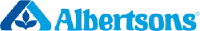Albertsons Companies Inc (NYSE:ACI)

-

-

FAQ

What is Discount Rate?

Discount rate reflects the opportunity cost of investment (i.e. the return that could be earned on investment with similar risk).

Cost of Equity Calculation

Cost of Equity
-
Rf
-
Beta
-
ERP
-

The Cost of Equity for Albertsons Companies Inc (NYSE:ACI) calculated via CAPM (Capital Asset Pricing Model) is -.

WACC Calculation

WACC
-

Cost of Equity
-
Equity Weight
-

Cost of Debt
-
Debt Weight
-

The WACC for Albertsons Companies Inc (NYSE:ACI) is -.